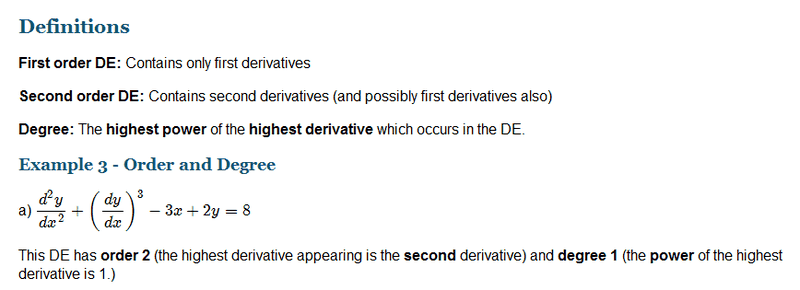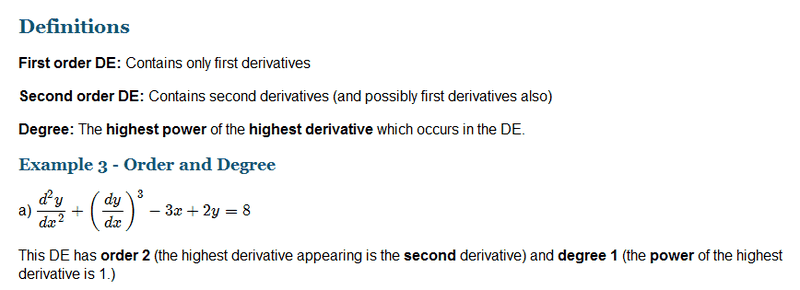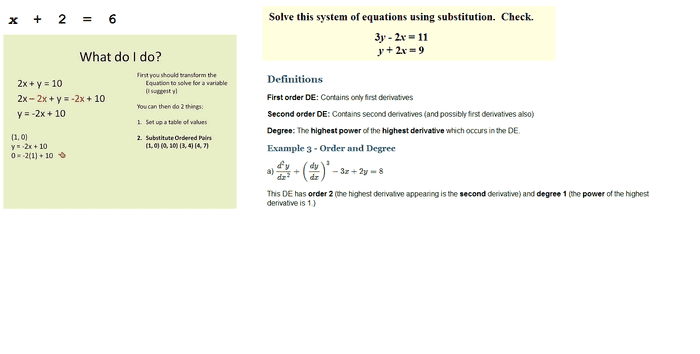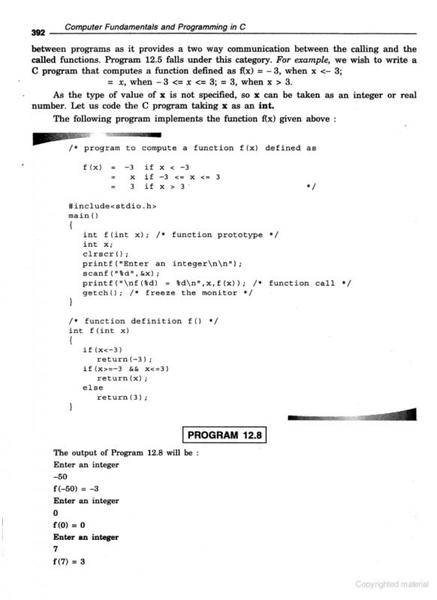# A few differential equation questions

i was trying to learn differential equations ...
i was lucky enough to find some good explanations for calculus and differential equations ...

The (standard) calculus is broken into two pieces.

i) Differential calculus - which is looking at the instantaneous rates of change of objects with respect to some variables. We have the notion of the derivative of a function.
ii) Integral calculus - which is calculating the area under curves, calculating volumes and so on. This is all given in terms if the (indefinite or definite) integral of a function.

The two notions are tied together via the fundamental theorem of calculus. This says that the derivative and indefinite integral are basically mutual inverses (but not quite)

Mod note: unrelated image now deleted
differential equations

first order differential equations
second order differential equations
higher order differential equations ...

linear differential equations
separable differential equations
exact differential equations
homogeneous differential equations
non homogeneous differential equations
using the method of undetermined coefficients ...

partial differential equations ...An equation containing the derivatives of one or more dependent variables, with respect to one or more independent variables, is said to be a differential equation

in this equation which is the dependent variables and which one is the independent variables ??

and how do i solve this differential equation ...

thanks ...

Last edited by a moderator:

The dependent variable changes with respect to the independent one, thus dependent = function of independent.

To solve the equation is to find a function of the independent variable, which has this special property that its second derivative + first derivative to the power of 3 - 3 times the independent variable + 2 times the function itself = 0.

To solve an ODE means to find the function as a clear expression or if it is hard to find one, you can use numerical methods to construct it. This is not easy and from what I see from your questions you should do more learning.

The dependent variable changes with respect to the independent one

so you mean when i separate these y term and x term ...

i should be able to get a dependent variable and an independent variable ??

To solve the equation is to find a function of the independent variable, which has this special property that its second derivative + first derivative to the power of 3 - 3 times the independent variable + 2 times the function itself = 0.

To solve an ODE means to find the function as a clear expression or if it is hard to find one, you can use numerical methods to construct it. This is not easy and from what I see from your questions you should do more learning.

yes i only started learning these ... few days ago ....

so you mean when i separate these y term and x term ...

i should be able to get a dependent variable and an independent variable ??

yes i only started learning these ... few days ago ....

Not always the case. You cannot always separate x and y from the given differential equation. The ones where you can separate x and y terms, are of the form
F(x)G(Y)dx+f(x)g(y)dy=0.

Ofcourse, it is also possible using a transformation or integrating factor. It is important to also know the forms of different differential equations etc. I would get Ross:Differential Equations.

yes ..i just figured out after few discussions here and there ... that differential equations are way more deeper than i first had in my mind ....

so i thought i would keep on talking about differential equations as much as i can ... and learn as much as i can with the help of discussions ...

so the first thing would be to ask few proper basic questions itself ...

The (standard) calculus is broken into two pieces.

i) Differential calculus - which is looking at the instantaneous rates of change of objects with respect to some variables. We have the notion of the derivative of a function.
ii) Integral calculus - which is calculating the area under curves, calculating volumes and so on. This is all given in terms if the (indefinite or definite) integral of a function.

The two notions are tied together via the http://cboard.cprogramming.com/redirect-to/?redirect=https%3A%2F%2Fen.wikipedia.org%2Fwiki%2FFundamental_theorem_of_calculus [Broken]. This says that the derivative and indefinite integral are basically mutual inverses (but not quite)

then , what is a differential equation ...

An equation containing the derivatives of one or more dependent variables, with respect to one or more independent variables, is said to be a differential equation

how many types of differential equations are there ??

first order differential equations
second order differential equations
higher order differential equations ...

linear differential equations
separable differential equations
exact differential equations
homogeneous differential equations
non homogeneous differential equations
using the method of undetermined coefficients ...

partial differential equations ...

what are the types of numerical methods associated with differential equations ...

Solution by Taylor's series
Picard's method of successive approximations
Euler's method
Modified Euler's Method
Runge-Kutta method
Predictor-Corrector Methods
Milne's method

how do you start solving the differential equations ??

i am not sure about this part ... because i have not tried to solve differential equations before ...

for example , for a polynomial ...

a solution of a polynomial equation is also called a root of the polynomial ...

a value for the variable that makes the polynomial zero

if you can't find an exact expression, then you can use numerical methods to get approximations ...

with numerical methods you can choose how close to zero you want, and it will give you a value that's at least that close ...

what is the best thing to do when you come across a differential equation question ??i personally think , one of the best things to do is to try to identify the type of differential equation in the question ...

and finally ...what sort of operations can be done on the differential equations ...??

Last edited by a moderator:
Better use of your time would be going through a differential equations book. I like Ross...

sure i will get that book somehow ...

thanks ...

sure i will get that book somehow ...

thanks ...
If you have not tried solving differential equations, then why think about them? Math is about getting one's hands dirty.

we had this subject in college called , "computer oriented numerical method in c programming language"

we were supposed to solve some maths with the help of numerical methods and c programming language ...

the syllabus sort of looked a bit like this ...

Solution of Algebraic and Transcendental Equation

2.1 Introduction
2.2 Bisection Method
2.3 Method of false position
2.4 Iteration method
2.5 Newton-Raphson Method
2.6 Ramanujan's method
2.7 The Secant Method
Finite Differences
3.1 Introduction
3.3.1 Forward differences
3.3.2 Backward differences
3.3.3 Central differences
3.3.4 Symbolic relations and separation of symbols
3.5 Differences of a polynomial

Interpolation

3.6 Newton's formulae for intrapolation
3.7 Central difference interpolation formulae
3.7.1 Gauss' Central Difference Formulae
3.9 Interpolation with unevenly spaced points
3.9.1 Langrange's interpolation formula
3.10 Divided differences and their properties
3.10.1 Newton's General interpolation formula
3.11 Inverse interpolation

Numerical Differentiation and Integration

5.1 Introduction
5.2 Numerical differentiation (using Newton's forward and backward formulae)
5.4 Numerical Integration
5.4.1 Trapizaoidal Rule
5.4.2 Simpson's 1/3-Rule
5.4.3 Simpson's 3/8-Rule

Matrices and Linear Systems of equations

6.3 Solution of Linear Systems – Direct Methods
6.3.2 Gauss elimination
6.3.3 Gauss-Jordan Method
6.3.4 Modification of Gauss method to compute the inverse
6.3.6 LU Decomposition
6.3.7 LU Decomposition from Gauss elimination
6.4 Solution of Linear Systems – Iterative methods
6.5 The eigen value problem
6.5.1 Eigen values of Symmetric Tridiazonal matrix

Numerical Solutions of Ordinary Differential Equations

7.1 Introduction
7.2 Solution by Taylor's series
7.3 Picard's method of successive approximations
7.4 Euler's method
7.4.2 Modified Euler's Method
7.5 Runge-Kutta method
7.6 Predictor-Corrector Methods
7.6.2 Milne's method

for example , for a polynomial ...

a solution of a polynomial equation is also called a root of the polynomial ...

a value for the variable that makes the polynomial zero

if you can't find an exact expression, then you can use numerical methods to get approximations ...

with numerical methods you can choose how close to zero you want, and it will give you a value that's at least that close ...

after all those , i had to move to computer programming in c language ...so it was important for me to ... declare terms like ... f(x) ... theta , d^2y/dx^2 , (dy/dx)^3... properly in a programming language like c ...

which was also why it was important to know what all those terms really meant ...

Last edited by a moderator:
that is why you read a differential equations book. Heck, even read Paul's Differential Notes if you do not have access for a book or find a free one on the internet. It is not hard to google. What you took seems to be a baby numerical analysis course..

we had this subject in college called , "computer oriented numerical method in c programming language"

we were supposed to solve some maths with the help of numerical methods and c programming language ...

the syllabus sort of looked a bit like this ...

after all those , i had to move to computer programming in c language ...so it was important for me to ... declare terms like ... f(x) ... theta , d^2y/dx^2 , (dy/dx)^3... properly in a programming language like c ...

which was also why it was important to know what all those terms really meant ...

I have to question your understanding on a few factors. How did you take this course without knowing what what derivatives are, what ordinary equations are, and how to solve them. Yet, your syllabus has such methods as Eulers, Picard, Runge Katta, and Interpolation?

Did you not read the requirements for the course? Or was is a class where the instructor assumed no prior knowledge and simplified his/her lectures?

If you want to understand what these terms, then read an ODE book. You will have understood what these things are in atleast 1 month of studying...

Last edited by a moderator:
mine was a three year Bsc , 6 semester computer science course ...

in the second semester , after some introduction to basic electronics ... we had this subject called ... "computer oriented numerical methods in c programming language "

the texts were only referring to the mathematics and the programming side of it was missing from most of the usual textbooks we had ...

the main programming language text ... The C Programming Language
The C Programming Language is a well-known computer programming book written by Brian Kernighan and Dennis Ritchie, the latter of whom originally designed and implemented the language
was missing numerical methods in it ...

so most people didn't had much clue either ... where this syllabus was heading to ...

most still doesn't have much clue ...

its only sometime later , for learning purposes ... i started to read these books instead ...

http://www.universityofcalicut.info/SDE/BSc_maths_numerical_methods.pdf

Computer Fundamentals and Programming in C - J.B. Dixit

i still don't have this book ... but the preview pages on google books looked way better than the original , The C Programming Language text book ...

i am clearly lacking materials to study these things ...

so i am still after book suggestions ...

does these belong to ODE ...
first order differential equations
second order differential equations
higher order differential equations ...

does these belong to the more advanced types of differential equations ...

linear differential equations
separable differential equations
exact differential equations
homogeneous differential equations
non homogeneous differential equations
using the method of undetermined coefficients ...

partial differential equations ...

??

Yes all belong to a differential equations for students who have no exposure to it. Some books have a partial differential sections, but it is better to get a separate book on that subject.

Do you live in the states?

i live in India ...

could you recommend a book for ODE ??

i live in India ...

could you recommend a book for ODE ??

I recommended Ross: Differential Equations. You can also google search differential books....

this is like \$10 only ...

i am going to get that book soon ...

thanks a lot ...

Mark44
Mentor
Better use of your time would be going through a differential equations book. I like Ross...
I agree 100%. The approach being taken by the OP is a waste of time IMO.

I think OP is missing the basic concepts and needs to catch up with them. When I stumble upon such problem, as already suggested, I use google to find an appropriate way to handle it.

From what I see in the syllabus maybe more appropriate book is something like this: https://www.math.ust.hk/~machas/differential-equations.pdf

Which is the second result in google when I searched "Introduction to ODE".

Good luck.

dpopchev ,

thanks ... i have a bit more better understanding of these terms now ...

i recommend this book too ...

William E Boyce , Richard C DiPrima - Elementary differential equations

anyway what i really did was .. collect many points from many books and made notes...

those with the help of things like directional fields and all ... helped in better understanding of differential equations ...

also this is a good place to start ... if you are lost ...

http://www.intmath.com/differential-equations/1-solving-des.php

thanks for the book suggestions ...